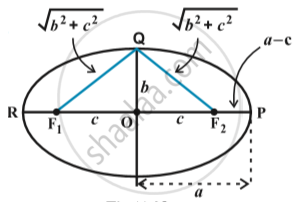CBSE (Arts) Class 11CBSE
Share

# Ellipse - Relationship Between Semi-major Axis, Semi-minor Axis and the Distance of the Focus from the Centre of the Ellipse

#### notesTake a point P at one end of the major axis.
Sum of the distances of the point P to the foci is
F_1 P + F_2P = F_1O + OP + F_2P (Since,  F_1P  = F_1O + OP) = c + a + a – c = 2a

Take a point Q at one end of the minor axis.
Sum of the distances from the point Q  to the foci is

F_1Q + F_2Q =  sqrt(b^2+c^2) + sqrt(b^2+c^2) = 2sqrt(b^2+c^2)

Since both P and Q lies on the  ellipse.
By the definition of ellipse, we have
2 sqrt(b^2+c^2) = 2a , i.e.,         a = sqrt(b^2+c^2)

or  a^2 = b^2 +c^2 , i.e.,               c = sqrt(a^2 - b^2)

S